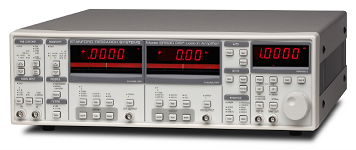﻿ Stanford Research Systems 830 Lock-in AmplifierSemiconductor Laboratory

## Stanford Research Systems 830 Lock-in AmplifierLock-in amplifiers can measure very small AC signals. Accurate measurements can be made even when the signal is obscured by a much larger noise signal. A lock-in amplifier uses an ac reference signal to drive a system and measures the response of the system only at the same frequency as the reference signal. Since noise is typically broadband (consisting of many frequencies), a lock-in amplifier can pick out a signal from the noise.

The frequency of the signal that will be measured can either be set by a separate frequency synthesizer or the internal reference signal of the SR830 can be used. There is a panel to control the internal reference signal on the right side of the instrument. Using the knob and buttons, the frequency, amplitude, and phase of the reference signal can be set. This reference signal is applied to a system to be measured and the response is input back to the lock-in amplifier at the signal input connectors on the left side of the instrument.

The input can either be a voltage applied to input A, a current applied to input A, or the difference in voltages applied to inputs A and B. The input signal $I(t)$ will contain the information we seek plus some noise. The component of the input signal at the frequency of the reference signal $f$ that is in-phase with the reference signal is projected out,

$$X(f)=\frac{2}{\tau}\int\limits_{t-\tau}^{t}\cos(2\pi f t')I(t')dt'.$$

Here $\tau$ is the time constant which can be selected in the upper left panel of the lock-in. The out-of-phase component is also projected out,

$$Y(f)=\frac{2}{\tau}\int\limits_{t-\tau}^{t}\sin(2\pi f t')I(t')dt'.$$

The amplitude $R$ and phase $\theta$ are related to the in-phase and out-of-phase components by,

$$R=\sqrt{X^2+Y^2},\qquad \tan (\theta) = \frac{Y}{X}.$$